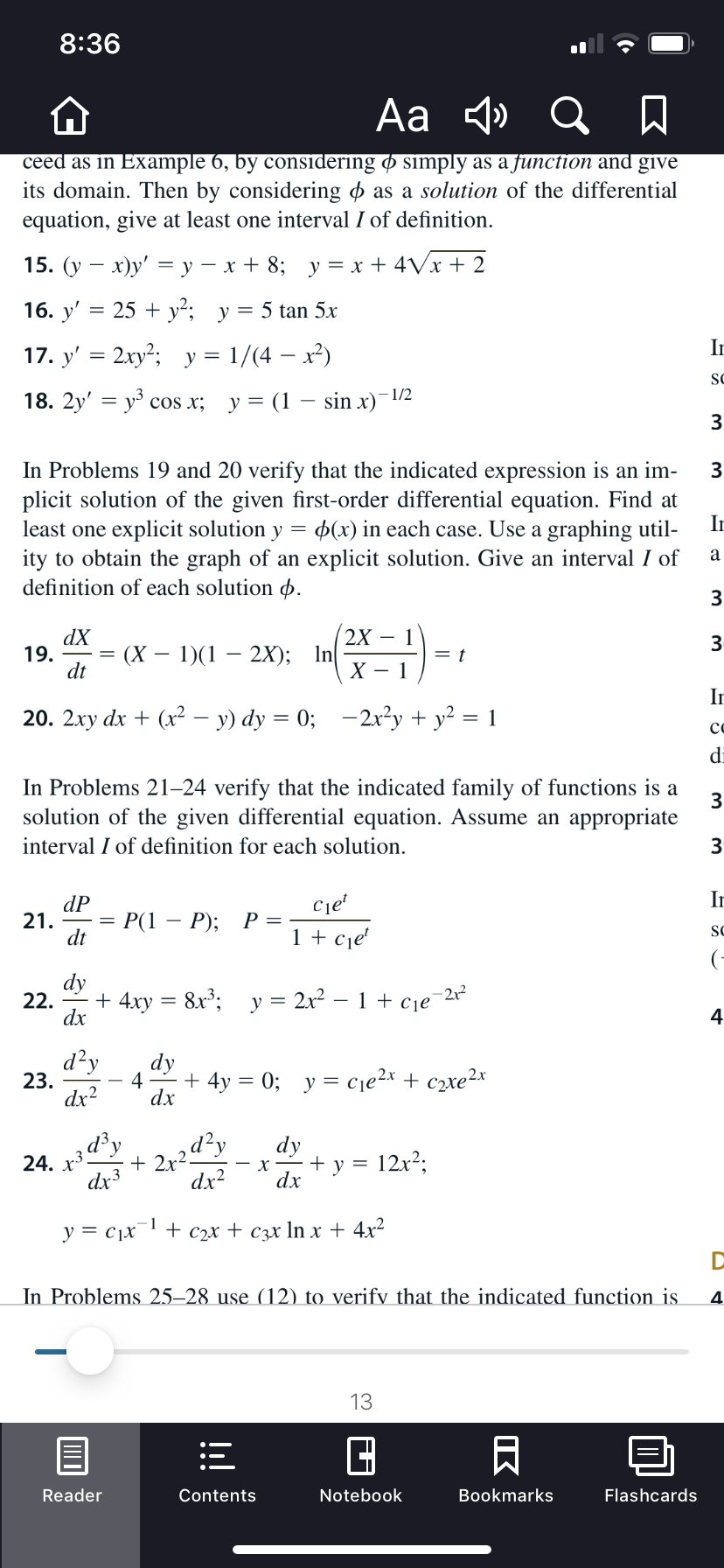# 8:36Aa 4» Q Aceed as in Example 6, by considering ø simply as a function and giveits domain. Then by considering o as a solution of the differentialequation, give at least one interval I of definition.15. (у — х)у' —у — х+ 8; у%3Dх + 4Vx+ 216. y' = 25 + y²; y= 5 tan 5xIn17. y' = 2xy²; y = 1/(4 – x²)SC- 1/218. 2y' — уз сos x; у%3D (1 — sin x)3In Problems 19 and 20 verify that the indicated expression is an im-plicit solution of the given first-order differential equation. Find atleast one explicit solution y = p(x) in each case. Use a graphing util-ity to obtain the graph of an explicit solution. Give an interval I ofdefinition of each solution p.In32X – 1dX19.dt(X – 1)(12X); InIn20. 2xy dx + (x2² – y) dy = 0; –2x²y + y² = 1codiIn Problems 21–24 verify that the indicated family of functions is asolution of the given differential equation. Assume an appropriateinterval I of definition for each solution.3IndP21.dtCe'P(1- P);1 + cje'SC(-dy22. - + 4xydx8x; y =2x² – 1 + cje-2124d?ydy4+ 4y = 0; y = cje2x + c2xe2x23.dx2dx* 2r2d?,dx?d³ydy12x2;24.dx3+ yхdx1y = c1x+ С2х + Сэх In x + 4x2In Problems 25–28 use (12) to verify that the indicated function is413ReaderNotebookBookmarksFlashcardsContentsIKШО

Question
4 views

#19help_outlineImage Transcriptionclose8:36 Aa 4» Q A ceed as in Example 6, by considering ø simply as a function and give its domain. Then by considering o as a solution of the differential equation, give at least one interval I of definition. 15. (у — х)у' —у — х+ 8; у%3Dх + 4Vx+ 2 16. y' = 25 + y²; y= 5 tan 5x In 17. y' = 2xy²; y = 1/(4 – x²) SC - 1/2 18. 2y' — уз сos x; у%3D (1 — sin x) 3 In Problems 19 and 20 verify that the indicated expression is an im- plicit solution of the given first-order differential equation. Find at least one explicit solution y = p(x) in each case. Use a graphing util- ity to obtain the graph of an explicit solution. Give an interval I of definition of each solution p. In 3 2X – 1 dX 19. dt (X – 1)(1 2X); In In 20. 2xy dx + (x2² – y) dy = 0; –2x²y + y² = 1 co di In Problems 21–24 verify that the indicated family of functions is a solution of the given differential equation. Assume an appropriate interval I of definition for each solution. 3 In dP 21. dt Ce' P(1 - P); 1 + cje' SC (- dy 22. - + 4xy dx 8x; y = 2x² – 1 + cje -212 4 d?y dy 4 + 4y = 0; y = cje2x + c2xe2x 23. dx2 dx * 2r2d?, dx? d³y dy 12x2; 24. dx3 + y х dx 1 y = c1x + С2х + Сэх In x + 4x2 In Problems 25–28 use (12) to verify that the indicated function is 4 13 Reader Notebook Bookmarks Flashcards Contents IK ШО fullscreen
check_circle

Step 1

Consider the function.

Step 2

Differentiate with respect to t.

Step 3

Take minus symbol outsid...

### Want to see the full answer?

See Solution

#### Want to see this answer and more?

Solutions are written by subject experts who are available 24/7. Questions are typically answered within 1 hour.*

See Solution
*Response times may vary by subject and question.
Tagged in

### Other